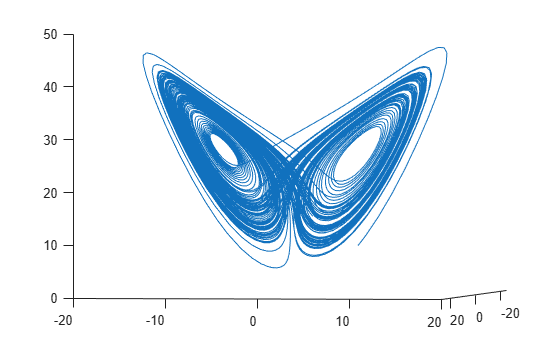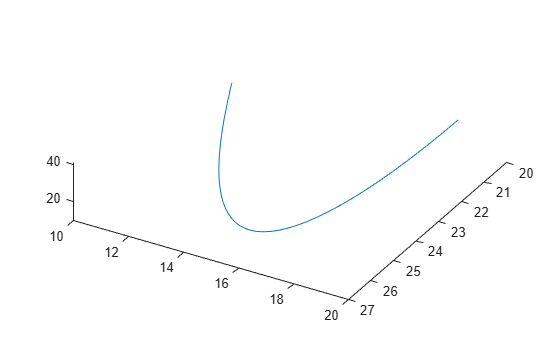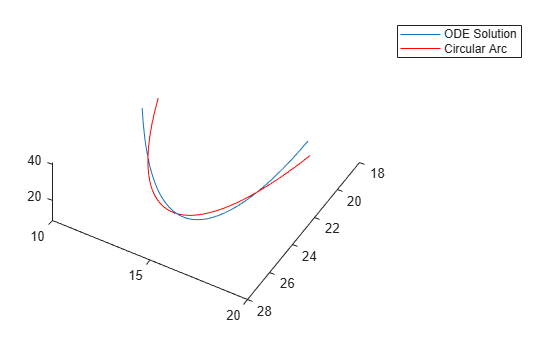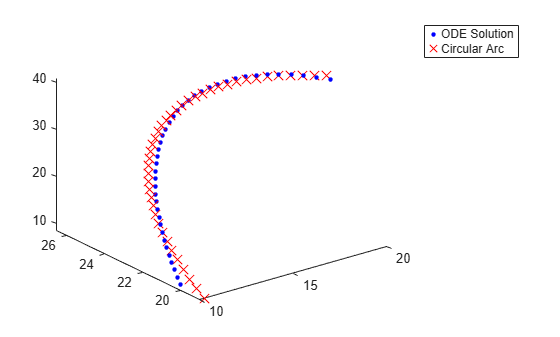## Fit an Ordinary Differential Equation (ODE)

This example shows how to fit parameters of an ODE to data in two ways. The first shows a straightforward fit of a constant-speed circular path to a portion of a solution of the Lorenz system, a famous ODE with sensitive dependence on initial parameters. The second shows how to modify the parameters of the Lorenz system to fit a constant-speed circular path. You can use the appropriate approach for your application as a model for fitting a differential equation to data.

### Lorenz System: Definition and Numerical Solution

The Lorenz system is a system of ordinary differential equations (see Lorenz system). For real constants $\sigma ,\phantom{\rule{0.5em}{0ex}}\rho ,\phantom{\rule{0.5em}{0ex}}\beta$, the system is

`$\begin{array}{l}\frac{dx}{dt}=\sigma \left(y-x\right)\\ \frac{dy}{dt}=x\left(\rho -z\right)-y\\ \frac{dz}{dt}=xy-\beta z.\end{array}$`

Lorenz's values of the parameters for a sensitive system are $\sigma =10,\phantom{\rule{0.5em}{0ex}}\beta =8/3,\phantom{\rule{0.5em}{0ex}}\rho =28$. Start the system from `[x(0),y(0),z(0)] = [10,20,10]` and view the evolution of the system from time 0 through 100.

```sigma = 10; beta = 8/3; rho = 28; f = @(t,a) [-sigma*a(1) + sigma*a(2); rho*a(1) - a(2) - a(1)*a(3); -beta*a(3) + a(1)*a(2)]; xt0 = [10,20,10]; [tspan,a] = ode45(f,[0 100],xt0); % Runge-Kutta 4th/5th order ODE solver figure plot3(a(:,1),a(:,2),a(:,3)) view([-10.0 -2.0])```The evolution is quite complicated. But over a small time interval, it looks somewhat like uniform circular motion. Plot the solution over the time interval `[0,1/10]`.

```[tspan,a] = ode45(f,[0 1/10],xt0); % Runge-Kutta 4th/5th order ODE solver figure plot3(a(:,1),a(:,2),a(:,3)) view([-30 -70])```### Fit a Circular Path to the ODE Solution

The equations of a circular path have several parameters:

• Angle $\theta \left(1\right)$ of the path from the x-y plane

• Angle $\theta \left(2\right)$ of the plane from a tilt along the x-axis

• Speed V

• Shift t0 from time 0

• 3-D shift in space delta

In terms of these parameters, determine the position of the circular path for times `xdata`.

`type fitlorenzfn`
```function f = fitlorenzfn(x,xdata) theta = x(1:2); R = x(3); V = x(4); t0 = x(5); delta = x(6:8); f = zeros(length(xdata),3); f(:,3) = R*sin(theta(1))*sin(V*(xdata - t0)) + delta(3); f(:,1) = R*cos(V*(xdata - t0))*cos(theta(2)) ... - R*sin(V*(xdata - t0))*cos(theta(1))*sin(theta(2)) + delta(1); f(:,2) = R*sin(V*(xdata - t0))*cos(theta(1))*cos(theta(2)) ... - R*cos(V*(xdata - t0))*sin(theta(2)) + delta(2); ```

To find the best-fitting circular path to the Lorenz system at times given in the ODE solution, use `lsqcurvefit`. In order to keep the parameters in reasonable limits, put bounds on the various parameters.

```lb = [-pi/2,-pi,5,-15,-pi,-40,-40,-40]; ub = [pi/2,pi,60,15,pi,40,40,40]; theta0 = [0;0]; R0 = 20; V0 = 1; t0 = 0; delta0 = zeros(3,1); x0 = [theta0;R0;V0;t0;delta0]; [xbest,resnorm,residual] = lsqcurvefit(@fitlorenzfn,x0,tspan,a,lb,ub);```
```Local minimum possible. lsqcurvefit stopped because the final change in the sum of squares relative to its initial value is less than the value of the function tolerance. ```

Plot the best-fitting circular points at the times from the ODE solution together with the solution of the Lorenz system.

```soln = a + residual; hold on plot3(soln(:,1),soln(:,2),soln(:,3),'r') legend('ODE Solution','Circular Arc') hold off``````figure plot3(a(:,1),a(:,2),a(:,3),'b.','MarkerSize',10) hold on plot3(soln(:,1),soln(:,2),soln(:,3),'rx','MarkerSize',10) legend('ODE Solution','Circular Arc') hold off```### Fit the ODE to the Circular Arc

Now modify the parameters $\sigma ,\phantom{\rule{0.5em}{0ex}}\beta ,\phantom{\rule{0.5em}{0ex}}and\phantom{\rule{0.5em}{0ex}}\rho$ to best fit the circular arc. For an even better fit, allow the initial point [10,20,10] to change as well.

To do so, write a function file `paramfun` that takes the parameters of the ODE fit and calculates the trajectory over the times `t`.

`type paramfun`
```function pos = paramfun(x,tspan) sigma = x(1); beta = x(2); rho = x(3); xt0 = x(4:6); f = @(t,a) [-sigma*a(1) + sigma*a(2); rho*a(1) - a(2) - a(1)*a(3); -beta*a(3) + a(1)*a(2)]; [~,pos] = ode45(f,tspan,xt0); ```

To find the best parameters, use `lsqcurvefit` to minimize the differences between the new calculated ODE trajectory and the circular arc `soln`.

```xt0 = zeros(1,6); xt0(1) = sigma; xt0(2) = beta; xt0(3) = rho; xt0(4:6) = soln(1,:); [pbest,presnorm,presidual,exitflag,output] = lsqcurvefit(@paramfun,xt0,tspan,soln);```
```Local minimum possible. lsqcurvefit stopped because the final change in the sum of squares relative to its initial value is less than the value of the function tolerance. ```

Determine how much this optimization changed the parameters.

`fprintf('New parameters: %f, %f, %f',pbest(1:3))`
```New parameters: 9.132446, 2.854998, 27.937986 ```
`fprintf('Original parameters: %f, %f, %f',[sigma,beta,rho])`
```Original parameters: 10.000000, 2.666667, 28.000000 ```

The parameters `sigma` and `beta` changed by about 10%.

Plot the modified solution.

```figure hold on odesl = presidual + soln; plot3(odesl(:,1),odesl(:,2),odesl(:,3),'b') plot3(soln(:,1),soln(:,2),soln(:,3),'r') legend('ODE Solution','Circular Arc') view([-30 -70]) hold off```### Problems in Fitting ODEs

As described in Optimizing a Simulation or Ordinary Differential Equation, an optimizer can have trouble due to the inherent noise in numerical ODE solutions. If you suspect that your solution is not ideal, perhaps because the exit message or exit flag indicates a potential inaccuracy, then try changing the finite differencing. In this example, use a larger finite difference step size and central finite differences.

```options = optimoptions('lsqcurvefit','FiniteDifferenceStepSize',1e-4,... 'FiniteDifferenceType','central'); [pbest2,presnorm2,presidual2,exitflag2,output2] = ... lsqcurvefit(@paramfun,xt0,tspan,soln,[],[],options);```
```Local minimum possible. lsqcurvefit stopped because the final change in the sum of squares relative to its initial value is less than the value of the function tolerance. ```

In this case, using these finite differencing options does not improve the solution.

`disp([presnorm,presnorm2])`
``` 20.0637 20.0637 ```

Watch now